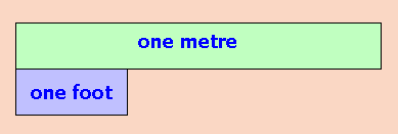Spike's Calculators

# Length Conversion Calculators

The International System of Units, abbreviated, SI (Système International), is the most widely used system of measurement. SI is the modern metric system of measurements. The metric system is a decimal-based system which makes it very simple to convert between units of measurement.

If SI is the modern metric system then the International Measures are the modern Imperial measurements.

The metre is the unit on which all length measurements are based.

```one metre =  3.28083989501312 feet
one foot = 1/ 3.28083989501312 = 0.3048 metre```

### SI, Metric Measures (Metric)

1 kilometre = 10 hectometres = 100 dekametres = 1000 metres = 10000 decimetres = 100000 centimetres = 1000000 millimetres

### International Measures (Imperial)

one international foot = 12 x 0.0254 metre (exactly) = 0.3048 metre
1 mile = 1760 yards = 5280 feet = 63360 inches

### US survey Measures

The United States uses the international and metric systems of measurements. In addition to that, measures derived from geodetic surveys within the United States in feet use the US survey foot for conversions to metres.
one survey foot = 1200/3937 metre (exactly) = 0.304800609601219 metre
one international foot = 0.0254 x 39.37 US survey foot (exactly) = 0.999 998 US survey footnot to scale